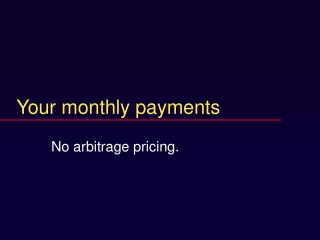DownloadDownload PresentationDownload Presentation- - - - - - - - - - - - - - - - - - - - - - - - - - - E N D - - - - - - - - - - - - - - - - - - - - - - - - - - -
##### Presentation Transcript

1. Your monthly payments No arbitrage pricing.

2. Key concepts • Real investment • Financial investment

3. Interest rate defined • Premium for current delivery

4. Basic principle • Firms maximize value • Owners maximize utility • Separately

5. Justification • Real investment with positive NPV shifts consumption opportunities outward. • Financial investment satisfies the owner’s time preferences.

7. Definitions • Coupon -- the amount paid periodically • Coupon rate -- the coupon times annual payments divided by 1000

8. Two parts of a bond • Principal paid at maturity. • A repeated constant flow -- an annuity

9. Strips • U.S. Treasury bonds • Stripped coupon is an annuity • Stripped principal is a payment of 1000 at maturity and nothing until then. • Stripped principal is also called a pure discount bond, a zero-coupon bond, or a zero, for short.

10. No arbitrage condition: • Price of bond = price of zero-coupon bond + price of stripped coupon. • Otherwise, a money machine, one way or the other. • Riskless increase in wealth

11. Pie theory • The bond is the whole pie. • The strip is one piece, the zero is the other. • Together, you get the whole pie. • No arbitrage pricing requires that the values of the pieces add up to the value of the whole pie.

12. Yogi Berra on finance • Cut my pizza in four slices, please. I’m not hungry enough for six.

13. Why use interest rates? • In addition to prices? • Answer: Coherence

14. Example: discount bonds • A zero pays 1000 at maturity. • Price (value) is the PV of that 1000 cash flow, using the market rate specific to the asset and maturity.

15. Example continued • Ten-year maturity: price is 426.30576 • Five-year maturity: price is 652.92095 • Similar or different? • They have the SAME discount rate (interest rate) r = .089 (i.e. 8.9%)

16. Calculations • 652.92095 = 1000 / (1+.089)5 • Note: ^ is spreadsheet notation for raising to a power • 426.30576 = 1000 / (1+.089)10

17. More realistically • For the ten-year discount bond, the price is 422.41081 (not 426.30576). • The ten-year rate is (1000/422.41081)1/10 - 1 = .09. • The 1/10 power is the tenth root. • It solves the equation 422.41081 = 1000/(1+r)10

18. Annuity • Interest rate per period, r. • Size of cash flows, C. • Maturity T. • If T=infinity, it’s called a perpetuity.

19. Market value of a perpetuity

20. Value of a perpetuity is C*(1/r) • In spreadsheet notation, * is the sign for multiplication. • Present Value of Perpetuity Factor, PVPF(r) = 1/r • It assumes that C = 1. • For any other C, multiply PVPF(r) by C.

21. Finished here 1/12/06

22. Value of an annuity • C (1/r)[1-1/(1+r)T] • Present value of annuity factor • PVAF(r,T) = (1/r)[1-1/(1+r)T] • or ATr

23. Explanation • Annuity = • difference in perpetuities. • One starts at time 1, • the other starts at time T + 1. • Value = difference in values (no arbitrage).

24. Explanation

25. Values • Value of the perpetuity starting at 1 is = 1/r … • in time zero dollars • Value of the perpetuity starting at T + 1 is = 1/r … • in time T dollars, • or (1/r)[1/(1+r)T] in time zero dollars. • Difference is PVAF(r,T)= (1/r)[1-1/(1+r)T]

26. Compounding • 12% is not 12% … ? • … when it is compounded.

27. E.A.R. Equivalent Annual rate

28. Example: which is better? • Wells Fargo: 8.3% compounded daily • World Savings: 8.65% uncompounded

29. Solution • Compare the equivalent annual rates • World Savings: EAR = .0865 • Wells Fargo: (1+.083/365)365 -1 = .0865314

30. Exam (sub) question • The interest rate is 6%, compounded monthly. • You set aside \$100 at the end of each month for 10 years. • How much money do you have at the end?

31. Answer Interest per period is .5% or .005. Present value is PVAF(120,.005)*100 = 9007.3451 Future value is 9007.3451*(1.005)120 = 16387.934